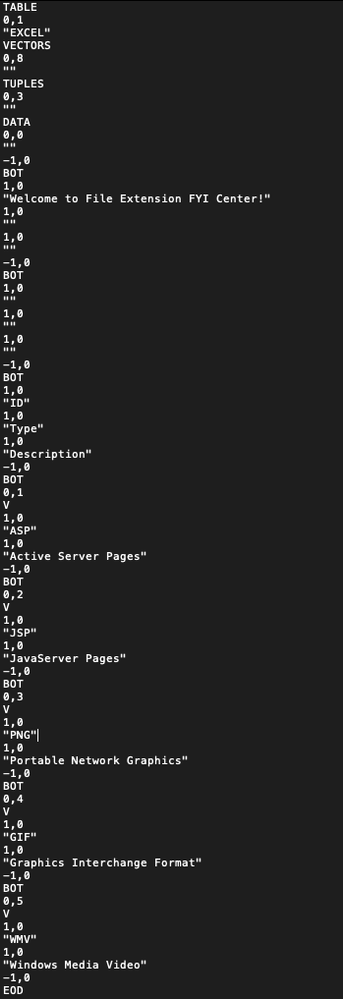stan_koprowskiCommunity Manager

## A basic text importer for Data Interchange Format (DIF) files

This add-in will create a JMP data table from a Data Interchange Format or DIF file.  The file format originated from Visicalc in the 1980 as a mechanism to transfer data between spreadsheet programs.

In the example code, I provide 4 mechanisms to check for the required headers with only one active at a time.

For additional information on Regex see @Craige_Hales' blog post and for insights into the Pat Match option see the JSL Cookbook.

By default, I have implemented Option 2: Pat Match for error checking.

1. Title
2. Vector
3. Tuples
4. Data

<< Attribute Name >>

<< Vector Number >> , << Numeric Value >>

<< "String value" >>

Where, |

<< Attribute_Name >> is a symbol/token of (usually) 32 or fewer characters

<< Vector Number >>  is 0 if entire file

<< Numeric Value >> is 0 unless otherwise specified

<< "String Value" >> is \!"\!" (double quote with no space between) if not used

1. Comment
2. Label
3. Size
4. Truelength
5. Units
6. DispalyUnits

DATA:

<< Data Type Indicator >>, << Numeric Value >>

<< "String value" >>

Where,

<< Data Type Indicator >> is one of three values -1, 0, 1

-1 --is a special data value

<< "String Value" >> = BOT or EOD

0 --numeric signed decimal number

<< Numeric Value >> = is numeric data

<< "String Value" >> = is one of the value indicators (V, NA, ERROR, TRUE, FALSE)

1 --string data value

<< Numeric Value >> = 0

<< "String Value" >> = string data value

``````errorhdrchkExpr = Expr(
regex_hdrs = "(TABLE|VECTORS|TUPLES|DATA)";
matrix_hdrs = { "TABLE", "VECTORS", "TUPLES", "DATA" };
rqd_hdrs = { TABLE, VECTORS, TUPLES, DATA };

//	Option 1: Find match using Regex with check for required headers
thematch = Regex( lines, regex_hdrs );
If( IsMissing(thematch),
errnum_desc = "Error: A required header is missing";
errhdrnum = 0;
Break();
,
errhdrnum = 1
);

//	Option 2: Find specific match using Pat Match with check for required headers
err_List = {};
For( e = 1, e <= Nitems( matrix_hdrs ), e++,
thematch = patMatch( txtfile, matrix_hdrs[e] );
If( thematch == 0,
errnum_desc = Concat("Error ", char( e ), ": ", "A required header ", matrix_hdrs[e], " is missing.");
Insert Into( err_List, errnum_desc);
errhdrnum = 0;
Break();
,
errhdrnum = 1
)
);

// Option 3: Find specific match using LOC with check for required headers
err_List = {};
For ( e = 1, e <= Nitems( rqd_hdrs ), e++,
If( Loc( {lines}, rqd_hdrs[e] ) != ,
errnum_desc = Concat("Error ", char( e ), ": ", "A required header ", rqd_hdrs[e], " is missing");
Insert Into( err_List, errnum_desc);
errhdrnum = 0;
Break();
,
errhdrnum = 1
)
);

// Option 4: Find specific match using Contains with check for required headers
err_List = {};
For ( e = 1, e <= Nitems( rqd_hdrs ), e++,
If( Contains( txtfile, rqd_hdrs[e] ) == 0,
errnum_desc = Concat("Error ", char( e ), ": ", "A required header ", rqd_hdrs[e], " is missing");
Insert Into( err_List, errnum_desc);
errhdrnum = 0;
Break();
,
errhdrnum = 1
)
);

);
``````

Sample.DIF file was used for the development and testing of the add-in.

The contents of the sample.dif file is shown in the attached image with the required headers contained.File Contents of sample.dif

The add-in was inspired by the community post from @JensRiege when he asked how to Import .dif file format in JMP Script.

cheers,

Stan

6 Stars! (out of 5)

I use this old file format daily since we have some legacy software systems.

Worked extremely well!

Thank you !!!

-Jens

Article Labels
Article Tags
Contributors